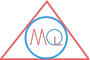# Fundamental Theorem of Arithmetic

## Theorem

Every number can be expressed as product of primes and this factorisation is unique, apart from the order in which the prime factors occur.

Example

45 is a composite number.

It is expressed as:

$$45 = 3 \times 3 \times 5$$

Note

Every number in maths can either be a prime or a composite number except 0 and 1 because 0 and 1 are neither a prime number nor a composite number.